Anúncio

# Unit 2 retest review

mlabuski
8 de Nov de 2012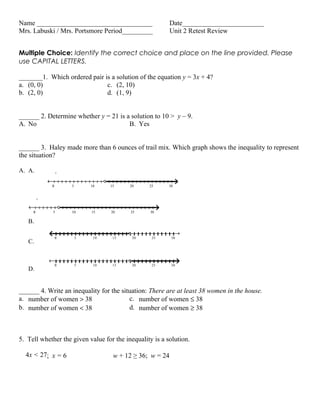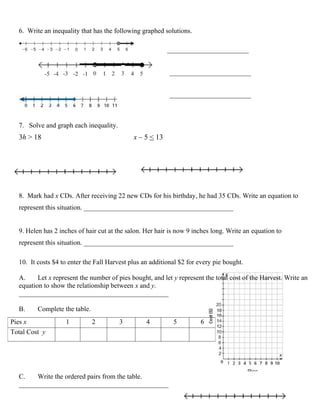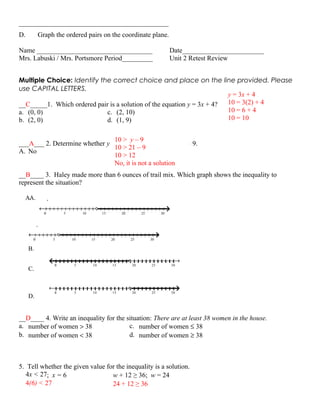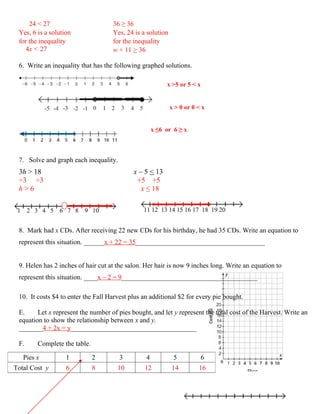Anúncio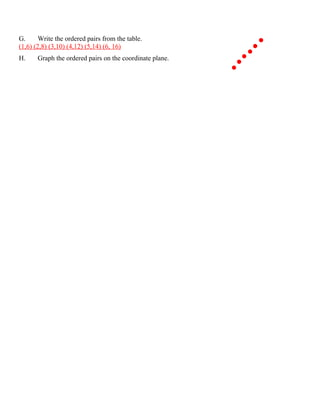Próximos SlideShares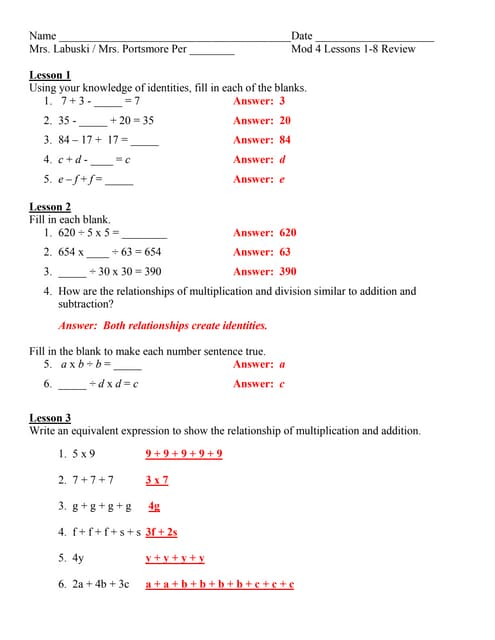Lesson 1 8 quiz review
Carregando em ... 3
1 de 5
Anúncio

### Unit 2 retest review

1. Name __________________________________ Date________________________ Mrs. Labuski / Mrs. Portsmore Period_________ Unit 2 Retest Review Multiple Choice: Identify the correct choice and place on the line provided. Please use CAPITAL LETTERS. _______1. Which ordered pair is a solution of the equation y = 3x + 4? a. (0, 0) c. (2, 10) b. (2, 0) d. (1, 9) ______ 2. Determine whether y = 21 is a solution to 10 > y – 9. A. No B. Yes ______ 3. Haley made more than 6 ounces of trail mix. Which graph shows the inequality to represent the situation? A. A. 0 5 10 15 20 25 30 0 5 10 15 20 25 30 B. 0 5 10 15 20 25 30 C. 0 5 10 15 20 25 30 D. ______ 4. Write an inequality for the situation: There are at least 38 women in the house. a. number of women > 38 c. number of women ≤ 38 b. number of women < 38 d. number of women ≥ 38 5. Tell whether the given value for the inequality is a solution. 4x < 27; x = 6 w + 12 ≥ 36; w = 24
2. 6. Write an inequality that has the following graphed solutions. __________________________ __________________________ __________________________ __________________________ 7. Solve and graph each inequality. 3h > 18 x – 5 < 13 8. Mark had x CDs. After receiving 22 new CDs for his birthday, he had 35 CDs. Write an equation to represent this situation. ____________________________________________ 9. Helen has 2 inches of hair cut at the salon. Her hair is now 9 inches long. Write an equation to represent this situation. ____________________________________________ 10. It costs \$4 to enter the Fall Harvest plus an additional \$2 for every pie bought. A. Let x represent the number of pies bought, and let y represent the total cost of the Harvest. Write an equation to show the relationship between x and y. ____________________________________________ B. Complete the table. Pies x 1 2 3 4 5 6 Total Cost y C. Write the ordered pairs from the table. ____________________________________________
3. ____________________________________________ D. Graph the ordered pairs on the coordinate plane. Name __________________________________ Date________________________ Mrs. Labuski / Mrs. Portsmore Period_________ Unit 2 Retest Review Multiple Choice: Identify the correct choice and place on the line provided. Please use CAPITAL LETTERS. y = 3x + 4 __C_____1. Which ordered pair is a solution of the equation y = 3x + 4? 10 = 3(2) + 4 a. (0, 0) c. (2, 10) 10 = 6 + 4 b. (2, 0) d. (1, 9) 10 = 10 10 > y – 9 ___A___ 2. Determine whether y = 21 is a solution to 10 > y – 9. 10 > 21 – 9 A. No B. Yes 10 > 12 No, it is not a solution __B____ 3. Haley made more than 6 ounces of trail mix. Which graph shows the inequality to represent the situation? A. . A 0 5 10 15 20 25 30 0 5 10 15 20 25 30 B. 0 5 10 15 20 25 30 C. 0 5 10 15 20 25 30 D. __D____ 4. Write an inequality for the situation: There are at least 38 women in the house. a. number of women > 38 c. number of women ≤ 38 b. number of women < 38 d. number of women ≥ 38 5. Tell whether the given value for the inequality is a solution. 4x < 27; x = 6 w + 12 ≥ 36; w = 24 4(6) < 27 24 + 12 ≥ 36
4. 24 < 27 36 ≥ 36 Yes, 6 is a solution Yes, 24 is a solution for the inequality for the inequality 4x < 27 w + 11 > 36 6. Write an inequality that has the following graphed solutions. x >5 or 5 < x x > 0 or 0 < x x ≤6 or 6 ≥ x __________________________ 7. Solve and graph each inequality. 3h > 18 x – 5 < 13 ÷3 ÷3 +5 +5 h>6 x ≤ 18 1 2 3 4 5 6 7 8 9 10 11 12 13 14 15 16 17 18 19 20 8. Mark had x CDs. After receiving 22 new CDs for his birthday, he had 35 CDs. Write an equation to represent this situation. ______x + 22 = 35______________________________________ 9. Helen has 2 inches of hair cut at the salon. Her hair is now 9 inches long. Write an equation to represent this situation. ____x – 2 = 9________________________________________ 10. It costs \$4 to enter the Fall Harvest plus an additional \$2 for every pie bought. E. Let x represent the number of pies bought, and let y represent the total cost of the Harvest. Write an equation to show the relationship between x and y. _______4 + 2x = y_____________________________________ F. Complete the table. Pies x 1 2 3 4 5 6 Total Cost y 6 8 10 12 14 16
5. G. Write the ordered pairs from the table. (1,6) (2,8) (3,10) (4,12) (5,14) (6, 16) H. Graph the ordered pairs on the coordinate plane.
Anúncio